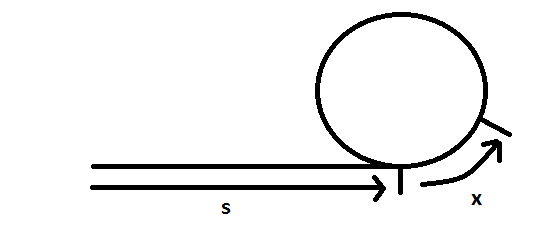With an inception in the year 1967, The Floyd’s cycle finding algorithm had been a commendable invention of Robert W Floyd, a computer scientist. By virtue of this algorithm, one can detect as well as identify the cycles from sequences which are stored in the structures of data or triggered on the fly. The most notable alternative of the Floyd’s Cycle Finding algorithm is the algorithm of the Pollard’s Rho which utilizes the process of integer factorization as well as the pseudo-random sequences for factoring integers. There’s as well an algorithm for the calculation of discrete logarithms on the basis of the Floyd’s Cycle finding algorithm.

Floyd’s Cycle Finding Algorithm is a part of C Programming

There is only 2 pointers used in the Floyd’s Cycle Finding algorithm which can move across the sequence at varied speeds. It is also denoted as the algorithm of tortoise and Hare enticing and accentuating the story of The tortoise and the Hare.

The cardinal implication of the algorithm is that in case of any integers i ≥ μ and k ≥ 0, xi = xi + kλ   in which λ  resembles the loop’s length  must be solved and the index of the first cycle’s element is μ. Hence, the algorithm shall just need to examine any repeated values of the special kind, one twice as far from the sequence’s start as the other in order to find the solution for period v which is a λ multiple. Post finding the solution for v, the algorithm has the sequence retraced from the beginning for finding the most preliminary repeated value xμ

In order to explain Floyd’s Algorithm, the below illustration and explanation shall be helpful.Consider that there are 2 positions:

S denotes the beginning of the cycle

S+x denotes wherein s represents the offset from the start of the cycle with the two pointers meeting.

After the slow pointer had to travel s+x, the faster one might have had travelled an extra distance denoted as s+x from the offset x position with the cycle. The additional s+x distance ensured to bring it back to the same state and position with the cycle called offset x.

This simply defines in case the distance s+x moved from the specific offset accompanies the pointer also to the offset x than having x moved lesser from that specific position, or so s shall have the outcome in the pointer being from the cycle’s start-position: offset 0

Hence, beginning the erstwhile fast pointer from where they had met (offset x with the cycle) and the erstwhile slow pointer right at the starting and migrating both those pointers with an equivalent space post s iterations, the erstwhile fast one shall be at the start of the cycle s and the erstwhile fast one shall also be at the start of the cycle s.Author:
Category :
Publish Date :
Social Sharing